Friday, October 22, 2021
Home > CBSE Class 10 > Circles Class 10 Maths MCQ with Answer Keys Solutions PDF DownloadCircles MCQ Questions for Class 10 Question No 11:

The angle between a tangent to a circle and the radius through the point of contact is

Option A : 60 degrees

Option B : 90 degrees

Option C : 120 degrees

Option D : 180 degrees

Option B : 90 degrees

Circles MCQ Questions for Class 10 Question No 12:

If two circles of radii 5 cm and 3 cm touch each other internally, then the distance between their centers is equal to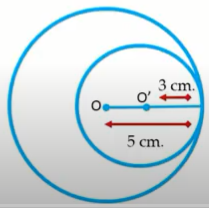Option A : 2

Option B : 3

Option C : 5

Option D : 8

Option A : 2

Circles MCQ Questions for Class 10 Question No 13:

Tangents PQ and PR are drawn to a circle from an external point P. If PQ = 9 cm and ∠PQR = 60o, then find the length of QR.Option A : 4.5 cm

Option B : 6 cm

Option C : 9 cm

Option D : 18 cm

Option C : 9 cm

Circles MCQ Questions for Class 10 Question No 14:

In the figure below, TA and TB are tangents drawn from an external point T. PQ is another tangent at S. If the perimeter of ∆PTQ is 20 cm, then the length of AT is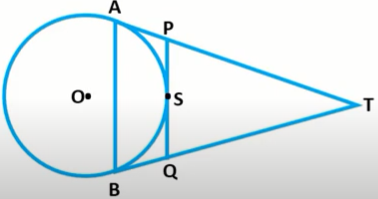Option A : 8 cm

Option B : 10 cm

Option C : 16 cm

Option D : 20 cm

Option B : 10 cm

Circles MCQ Questions for Class 10 Question No 15:

In figure below, AC, CE and EH are tangents drawn to the circle at B, D and F respectively. If CB = 5 cm, EF = 3 cm, then the length of CE is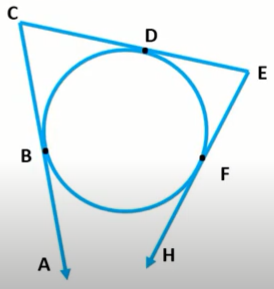Option A : 2 cm

Option B : 5 cm

Option C : 3 cm

Option D : 8 cm

Option D : 8 cm

Circles MCQ Questions for Class 10 Question No 16:

In figure below, if ∠PAO = 30o, then find the angle ∠POQ.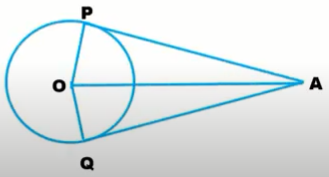Option A : 60o

Option B : 120o

Option C : 90o

Option D : 30o

Option B : 120o

Circles MCQ Questions for Class 10 Question No 17:

In the figure below, O is the centre of the circle, AB is a chord and AT is the tangent at A. If ∠AOB = 100o, then find the angle ∠BAT.Option A : 40o

Option B : 60o

Option C : 70o

Option D : 50o

Option D : 50o

Circles MCQ Questions for Class 10 Question No 18:

In the figure below, AB, AC and AD are tangents. If AB = 5 cm, then find the length of AD.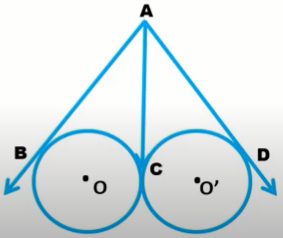Option A : 4

Option B : 3

Option C : 5

Option D : 6

Option C : 5

Circles MCQ Questions for Class 10 Question No 19:

Two concentric circles are of radii 5 cm and 3 cm. The length of the chord of the larger circle which touches the smaller circle is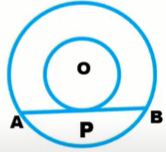Option A : 8

Option B : 10

Option C : 12

Option D : 18

Option A : 8

Circles MCQ Questions for Class 10 Question No 20:

In the figure below, if TP and TQ are the two tangents to a circle with the center O so that ∠POQ = 110 degrees, then find the value of the angle ∠PTQ.Option A : 60o

Option B : 70o

Option C : 80o

Option D : 90o